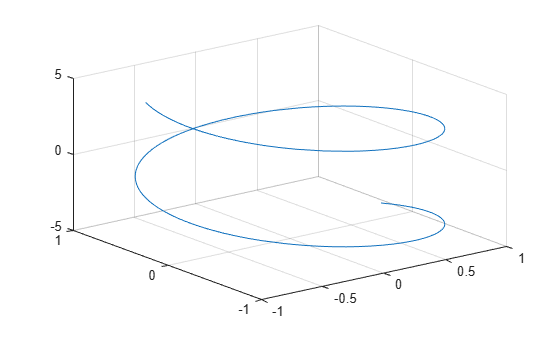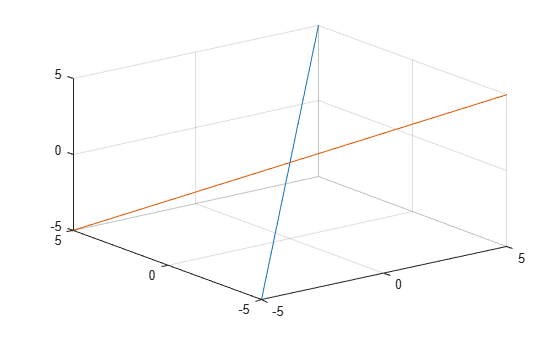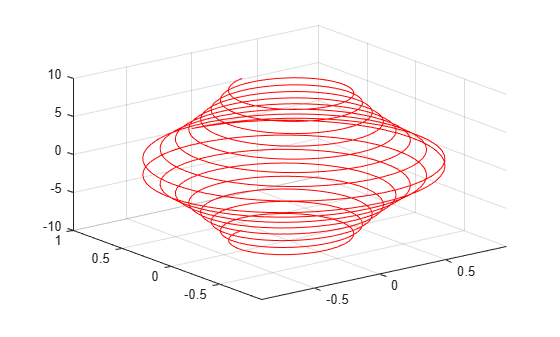Main Content

# fplot3

3-D parametric curve plotter

## Syntax

fplot3(funx,funy,funz)
fplot3(funx,funy,funz,tinterval)
fplot3(___,LineSpec)
fplot3(___,Name,Value)
fplot3(ax,___)
fp = fplot3(___)

## Description

example

fplot3(funx,funy,funz) plots the parametric curve defined by x = funx(t), y = funy(t), and z = funz(t) over the default interval [-5,5] for t.

example

fplot3(funx,funy,funz,tinterval) plots over the specified interval. Specify the interval as a two-element vector of the form [tmin tmax].

example

fplot3(___,LineSpec) sets the line style, marker symbol, and line color. For example, '-r' specifies a red line. Use this option after any of the previous input argument combinations.
fplot3(___,Name,Value) specifies line properties using one or more name-value pair arguments. For example, 'LineWidth',2 specifies a line width of 2 points.
fplot3(ax,___) plots into the axes specified by ax instead of the current axes. Specify the axes as the first input argument.

example

fp = fplot3(___) returns a ParameterizedFunctionLine object. Use the object to query and modify properties of a specific line. For a list of properties, see ParameterizedFunctionLine Properties.

## Examples

collapse all

Plot the 3-D parametric line

$\begin{array}{c}x=\mathrm{sin}\left(t\right)\\ y=\mathrm{cos}\left(t\right)\\ z=t\end{array}$

over the default parameter range [-5 5].

xt = @(t) sin(t); yt = @(t) cos(t); zt = @(t) t; fplot3(xt,yt,zt)Plot the parametric line

$\begin{array}{c}x={e}^{-t/10}\mathrm{sin}\left(5t\right)\\ y={e}^{-t/10}\mathrm{cos}\left(5t\right)\\ z=t\end{array}$

over the parameter range [-10 10] by specifying the fourth input argument of fplot3.

xt = @(t) exp(-t/10).*sin(5*t); yt = @(t) exp(-t/10).*cos(5*t); zt = @(t) t; fplot3(xt,yt,zt,[-10 10])Plot the same 3-D parametric curve three times over different intervals of the parameter. For the first interval, use a line width of 2 points. For the second, specify a dashed red line style with circle markers. For the third, specify a cyan, dash-dotted line style with asterisk markers.

fplot3(@(t)sin(t), @(t)cos(t), @(t)t, [0 2*pi], 'LineWidth', 2) hold on fplot3(@(t)sin(t), @(t)cos(t), @(t)t, [2*pi 4*pi], '--or') fplot3(@(t)sin(t), @(t)cos(t), @(t)t, [4*pi 6*pi], '-.*c') hold offPlot multiple lines in the same axes using hold on.

fplot3(@(t)t, @(t)t, @(t)t) hold on fplot3(@(t)-t, @(t)t, @(t)-t) hold offPlot the parametric line

$\begin{array}{c}x={e}^{-|t|/10}\mathrm{sin}\left(5|t|\right)\\ y={e}^{-|t|/10}\mathrm{cos}\left(5|t|\right)\\ z=t.\end{array}$

Assign the parameterized function line object to a variable.

xt = @(t)exp(-abs(t)/10).*sin(5*abs(t)); yt = @(t)exp(-abs(t)/10).*cos(5*abs(t)); zt = @(t)t; fp = fplot3(xt,yt,zt)fp = ParameterizedFunctionLine with properties: XFunction: @(t)exp(-abs(t)/10).*sin(5*abs(t)) YFunction: @(t)exp(-abs(t)/10).*cos(5*abs(t)) ZFunction: @(t)t Color: [0 0.4470 0.7410] LineStyle: '-' LineWidth: 0.5000 Show all properties 

Change the range of parameter values to [-10 10] and change the line color to red.

fp.TRange = [-10 10]; fp.Color = 'r';For $t$ values in the range $-2\pi$ to $2\pi$, plot the parametric line

$\begin{array}{c}x=t\\ y=t/2\\ z=\mathrm{sin}\left(6t\right).\end{array}$

Add a title, x-axis label, and y-axis label. Additionally, change the view of the axes and display the axes box outline.

xt = @(t)t; yt = @(t)t/2; zt = @(t)sin(6*t); fplot3(xt,yt,zt,[-2*pi 2*pi],'MeshDensity',30,'LineWidth',1); title('x=t, y=t/2, z=sin(6t) for -2\pi<t<2\pi') xlabel('x'); ylabel('y'); view(52.5,30) box onAccess the axes object using gca. Specify the x-axis tick values and associated labels using the XTick and XTickLabel properties of the axes object. Similarly, specify the y-axis tick values and associated labels.

ax = gca; ax.XTick = -2*pi:pi/2:2*pi; ax.XTickLabel = {'-2\pi','-3\pi/2','-\pi','-\pi/2','0','\pi/2','\pi','3\pi/2','2\pi'}; ax.YTick = -pi:pi/2:pi; ax.YTickLabel = {'-\pi','-\pi/2','0','\pi/2','\pi'};## Input Arguments

collapse all

Parametric function for x coordinates, specified as a function handle to a named or anonymous function.

Specify a function of the form x = funx(t). The function must accept a vector input argument and return a vector output argument of the same size. Use array operators instead of matrix operators for the best performance. For example, use .* (times) instead of * (mtimes).

Example: funx = @(t) sin(2*t);

Parametric function for y coordinates, specified as a function handle to a named or anonymous function.

Specify a function of the form y = funy(t). The function must accept a vector input argument and return a vector output argument of the same size. Use array operators instead of matrix operators for the best performance. For example, use .* (times) instead of * (mtimes).

Example: funy = @(t) cos(2*t);

Parametric function for z coordinates, specified as a function handle to a named or anonymous function.

Specify a function of the form z = funz(t). The function must accept a vector input argument and return a vector output argument of the same size. Use array operators instead of matrix operators for the best performance. For example, use .* (times) instead of * (mtimes).

Example: funz = @(t) t;

Interval for parameter t, specified as a two-element vector of the form [tmin tmax].

Axes object. If you do not specify an axes object, then fplot3 uses the current axes (gca).

Line style, marker, and color, specified as a character vector or string containing symbols. The symbols can appear in any order. You do not need to specify all three characteristics (line style, marker, and color). For example, if you omit the line style and specify the marker, then the plot shows only the marker and no line.

Example: '--or' is a red dashed line with circle markers

Line StyleDescription
-Solid line
--Dashed line
:Dotted line
-.Dash-dot line
MarkerDescription
'o'Circle
'+'Plus sign
'*'Asterisk
'.'Point
'x'Cross
'_'Horizontal line
'|'Vertical line
's'Square
'd'Diamond
'^'Upward-pointing triangle
'v'Downward-pointing triangle
'>'Right-pointing triangle
'<'Left-pointing triangle
'p'Pentagram
'h'Hexagram
ColorDescription

y

yellow

m

magenta

c

cyan

r

red

g

green

b

blue

w

white

k

black

### Name-Value Pair Arguments

Specify optional comma-separated pairs of Name,Value arguments. Name is the argument name and Value is the corresponding value. Name must appear inside quotes. You can specify several name and value pair arguments in any order as Name1,Value1,...,NameN,ValueN.

Example: 'Marker','o','MarkerFaceColor','red'

The properties listed here are only a subset. For a complete list, see ParameterizedFunctionLine Properties.

Number of evaluation points, specified as a number. The default is 23. Because fplot3 uses adaptive evaluation, the actual number of evaluation points is greater.

Line color, specified as an RGB triplet, a hexadecimal color code, a color name, or a short name.

For a custom color, specify an RGB triplet or a hexadecimal color code.

• An RGB triplet is a three-element row vector whose elements specify the intensities of the red, green, and blue components of the color. The intensities must be in the range [0,1]; for example, [0.4 0.6 0.7].

• A hexadecimal color code is a character vector or a string scalar that starts with a hash symbol (#) followed by three or six hexadecimal digits, which can range from 0 to F. The values are not case sensitive. Thus, the color codes '#FF8800', '#ff8800', '#F80', and '#f80' are equivalent.

Alternatively, you can specify some common colors by name. This table lists the named color options, the equivalent RGB triplets, and hexadecimal color codes.

Color NameShort NameRGB TripletHexadecimal Color CodeAppearance
'red''r'[1 0 0]'#FF0000''green''g'[0 1 0]'#00FF00''blue''b'[0 0 1]'#0000FF''cyan' 'c'[0 1 1]'#00FFFF''magenta''m'[1 0 1]'#FF00FF''yellow''y'[1 1 0]'#FFFF00''black''k'[0 0 0]'#000000''white''w'[1 1 1]'#FFFFFF'Here are the RGB triplets and hexadecimal color codes for the default colors MATLAB® uses in many types of plots.

RGB TripletHexadecimal Color CodeAppearance
[0 0.4470 0.7410]'#0072BD'[0.8500 0.3250 0.0980]'#D95319'[0.9290 0.6940 0.1250]'#EDB120'[0.4940 0.1840 0.5560]'#7E2F8E'[0.4660 0.6740 0.1880]'#77AC30'[0.3010 0.7450 0.9330]'#4DBEEE'[0.6350 0.0780 0.1840]'#A2142F'Example: 'blue'

Example: [0 0 1]

Example: '#0000FF'

Line style, specified as one of the options listed in this table.

Line StyleDescriptionResulting Line
'-'Solid line'--'Dashed line':'Dotted line'-.'Dash-dotted line'none'No lineNo line

Line width, specified as a positive value in points, where 1 point = 1/72 of an inch. If the line has markers, then the line width also affects the marker edges.

The line width cannot be thinner than the width of a pixel. If you set the line width to a value that is less than the width of a pixel on your system, the line displays as one pixel wide.

Marker symbol, specified as one of the values listed in this table. By default, the object does not display markers. Specifying a marker symbol adds markers at each data point or vertex.

ValueDescription
'o'Circle
'+'Plus sign
'*'Asterisk
'.'Point
'x'Cross
'_'Horizontal line
'|'Vertical line
'square' or 's'Square
'diamond' or 'd'Diamond
'^'Upward-pointing triangle
'v'Downward-pointing triangle
'>'Right-pointing triangle
'<'Left-pointing triangle
'pentagram' or 'p'Five-pointed star (pentagram)
'hexagram' or 'h'Six-pointed star (hexagram)
'none'No markers

Marker outline color, specified as 'auto', an RGB triplet, a hexadecimal color code, a color name, or a short name. The default value of 'auto' uses the same color as the Color property.

For a custom color, specify an RGB triplet or a hexadecimal color code.

• An RGB triplet is a three-element row vector whose elements specify the intensities of the red, green, and blue components of the color. The intensities must be in the range [0,1]; for example, [0.4 0.6 0.7].

• A hexadecimal color code is a character vector or a string scalar that starts with a hash symbol (#) followed by three or six hexadecimal digits, which can range from 0 to F. The values are not case sensitive. Thus, the color codes '#FF8800', '#ff8800', '#F80', and '#f80' are equivalent.

Alternatively, you can specify some common colors by name. This table lists the named color options, the equivalent RGB triplets, and hexadecimal color codes.

Color NameShort NameRGB TripletHexadecimal Color CodeAppearance
'red''r'[1 0 0]'#FF0000''green''g'[0 1 0]'#00FF00''blue''b'[0 0 1]'#0000FF''cyan' 'c'[0 1 1]'#00FFFF''magenta''m'[1 0 1]'#FF00FF''yellow''y'[1 1 0]'#FFFF00''black''k'[0 0 0]'#000000''white''w'[1 1 1]'#FFFFFF''none'Not applicableNot applicableNot applicableNo color

Here are the RGB triplets and hexadecimal color codes for the default colors MATLAB uses in many types of plots.

RGB TripletHexadecimal Color CodeAppearance
[0 0.4470 0.7410]'#0072BD'[0.8500 0.3250 0.0980]'#D95319'[0.9290 0.6940 0.1250]'#EDB120'[0.4940 0.1840 0.5560]'#7E2F8E'[0.4660 0.6740 0.1880]'#77AC30'[0.3010 0.7450 0.9330]'#4DBEEE'[0.6350 0.0780 0.1840]'#A2142F'Marker fill color, specified as 'auto', an RGB triplet, a hexadecimal color code, a color name, or a short name. The 'auto' value uses the same color as the MarkerEdgeColor property.

For a custom color, specify an RGB triplet or a hexadecimal color code.

• An RGB triplet is a three-element row vector whose elements specify the intensities of the red, green, and blue components of the color. The intensities must be in the range [0,1]; for example, [0.4 0.6 0.7].

• A hexadecimal color code is a character vector or a string scalar that starts with a hash symbol (#) followed by three or six hexadecimal digits, which can range from 0 to F. The values are not case sensitive. Thus, the color codes '#FF8800', '#ff8800', '#F80', and '#f80' are equivalent.

Alternatively, you can specify some common colors by name. This table lists the named color options, the equivalent RGB triplets, and hexadecimal color codes.

Color NameShort NameRGB TripletHexadecimal Color CodeAppearance
'red''r'[1 0 0]'#FF0000''green''g'[0 1 0]'#00FF00''blue''b'[0 0 1]'#0000FF''cyan' 'c'[0 1 1]'#00FFFF''magenta''m'[1 0 1]'#FF00FF''yellow''y'[1 1 0]'#FFFF00''black''k'[0 0 0]'#000000''white''w'[1 1 1]'#FFFFFF''none'Not applicableNot applicableNot applicableNo color

Here are the RGB triplets and hexadecimal color codes for the default colors MATLAB uses in many types of plots.

RGB TripletHexadecimal Color CodeAppearance
[0 0.4470 0.7410]'#0072BD'[0.8500 0.3250 0.0980]'#D95319'[0.9290 0.6940 0.1250]'#EDB120'[0.4940 0.1840 0.5560]'#7E2F8E'[0.4660 0.6740 0.1880]'#77AC30'[0.3010 0.7450 0.9330]'#4DBEEE'[0.6350 0.0780 0.1840]'#A2142F'Example: [0.3 0.2 0.1]

Example: 'green'

Example: '#D2F9A7'

Marker size, specified as a positive value in points, where 1 point = 1/72 of an inch.

## Output Arguments

collapse all

One or more ParameterizedFunctionLine objects, returned as a scalar or a vector. You can use these objects to query and modify properties of a specific ParameterizedFunctionLine object. For details, see ParameterizedFunctionLine Properties.

Introduced in R2016a

Download ebook